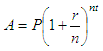# Calculate Compound Interest

Below you can find a spreadsheet to calculate compound interest easily...

Compound interest appears when interest is added to the principal, so that from that instant on, the interest that has been added earns interest, too. The addition to the principal is called compounding. For example, an ordinary bank account may have its interest compounded every year. In that case, an account with \$100 initial principal and 10% interest per year would have a balance of \$110 at the end of the first year, and \$121 at the end of the second year.

In order to define an interest rate fully, the interest rate and the compounding frequency must be disclosed. Compound interest may be compared with simple interest, where interest isn't added to the principal.
Compound interest is standard in finance and economics

The compound interest formula is given by:where
A = amount, or ending balance
P = principal
r = annual interest rate
n = compounded times per year
t = number of years

### Continuous compounding

Continuous compounding is another concept that can be thought of as making the compounding period infinitesimally small.

This formula works is:

A = P ert

where

A = ending balance
P = principal
e = 2.718281...
t = number of years
r = annual interest rate

### Example:

Find the amount of an investment if \$1,000 is invested at 5.6% compounded monthly for four years.

We enter our data in the calculator below...

Principal = 1,000.00
Interest rate per yr = 5.60%
Compounded times per yr = 12
Time in years = 4

And we get our results

Amount = 1,250.42
With continuously compound interest = 1,251.07

To be able to use the following calculator you have to allow the use of JavaScript codes on your navigator.

### Compound Interest Calculator

 Enter the needed values...(don't use commas) Principal: Interest Rate per Year: % Compounded times per year: Time in years: Amount: With continuously compound interest:

From 'Calculate Compound Interest' to home

From 'Calculate Compound Interest' to Free Online Calculators